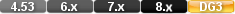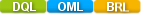Easy to Create, Easy to Change - Easy to use!## JulianJulian(DATE VALUE) Julian(Current Date) Julian(24/12/12)

The julian function converts a date value to a Julian date.

YY are the last two digits of the year. DDD is the position of the day in the year (with January 1st = 001; and December 31 = 365, except in leap year when it is 366).

# Parameters

DATE VALUE

Any DataEase Date Value or date formatted constant.

# Returns/Result

A five-digit integer in the format YYDDD.

# Examples

Examples

julian( 07/04/99)

Returns: 99185

julian( 12/31/99)

Returns: 99365

# julian

Date Function

#### Purpose

The julian function converts a date value to a Julian date.

Syntax

julian( DATE VALUE)

Returns

A five-digit integer in the format YYDDD.

Usage

YY are the last two digits of the year. DDD is the position of the day in the year (with January 1st = 001; and December 31 = 365, except in leap year when it is 366).

Examples

julian( 07/04/99)

Returns: 99185

julian( 12/31/99)

Returns: 99365